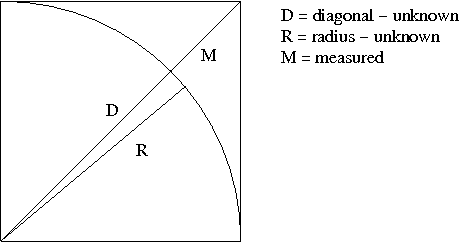# Spa Corner Radius MeasurementHere is an alternative way to measure the radius of the corner of a Spa. Most guides recommend putting a square up to the corner of the Spa, then judging the point where the streight edge touches the square. However as soon as you look at that, you can see that a 1mm difference in judging 'touch' can change the radius by 20mm. As you require a square any how it dawned on me that a much easier measurement to take is from the corner of the square to the radius of the curve. Even if you don't get the angle for measuring M correct, there will be little difference in the value you measure.

To cut a long story short,  Radius = 2.414 * Measurement

The long story for those that care, or those that never thought high school maths would be useful in later life.

From pythagoras we know that D and R are related

D2 = R2 + R2

We also can observe that

D = R + M

So combining these we get

R2 + R2 = ( R + M ) 2

Expand and re-arrange to standard quadratic format
R2 + R2 = R2 + 2 M R + M2
R2 - 2MR - M2 = 0

Now this can be solved for R by using the quadratic formulaWhere:
a = 1 (As it is always multiplied by you can ignore it)
b = -2M
c = - M2

So
R = ( -2M + √4M2 - (- 4 M2)   ) / 2
R = ( -2M + √8M2  ) / 2
R = ( -2M + 2√2 M ) / 2
R = M + √2 M

To make it even simpler, to 4 digits 1+√2 is 2.414 so with any calculator you can find
R = 2.414 * M